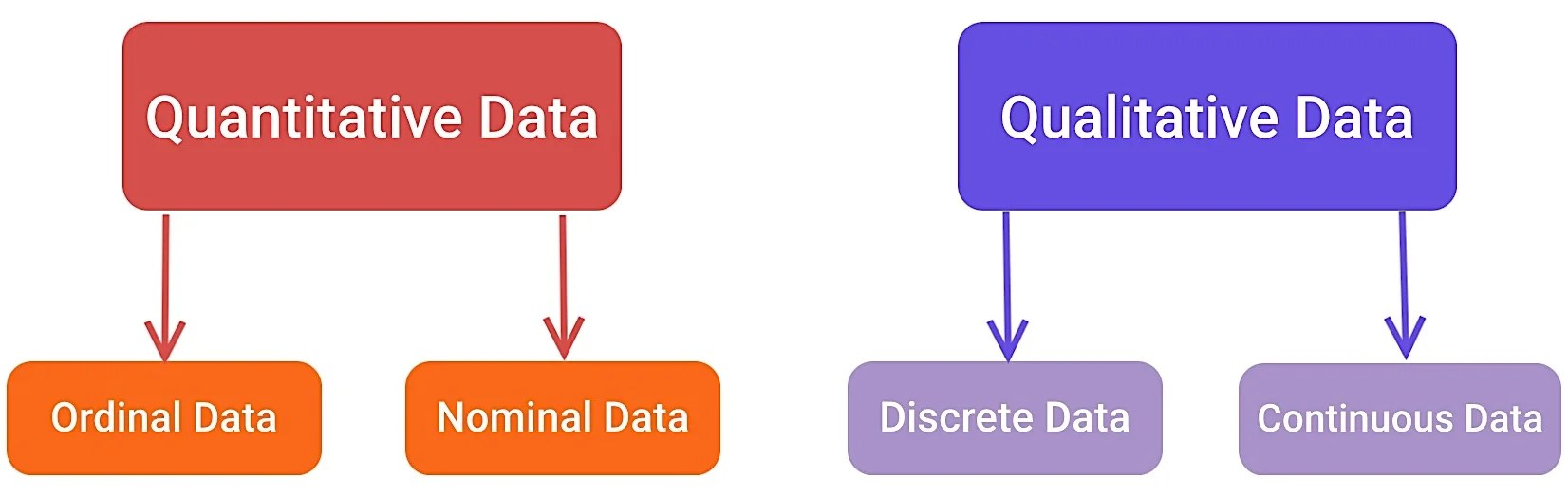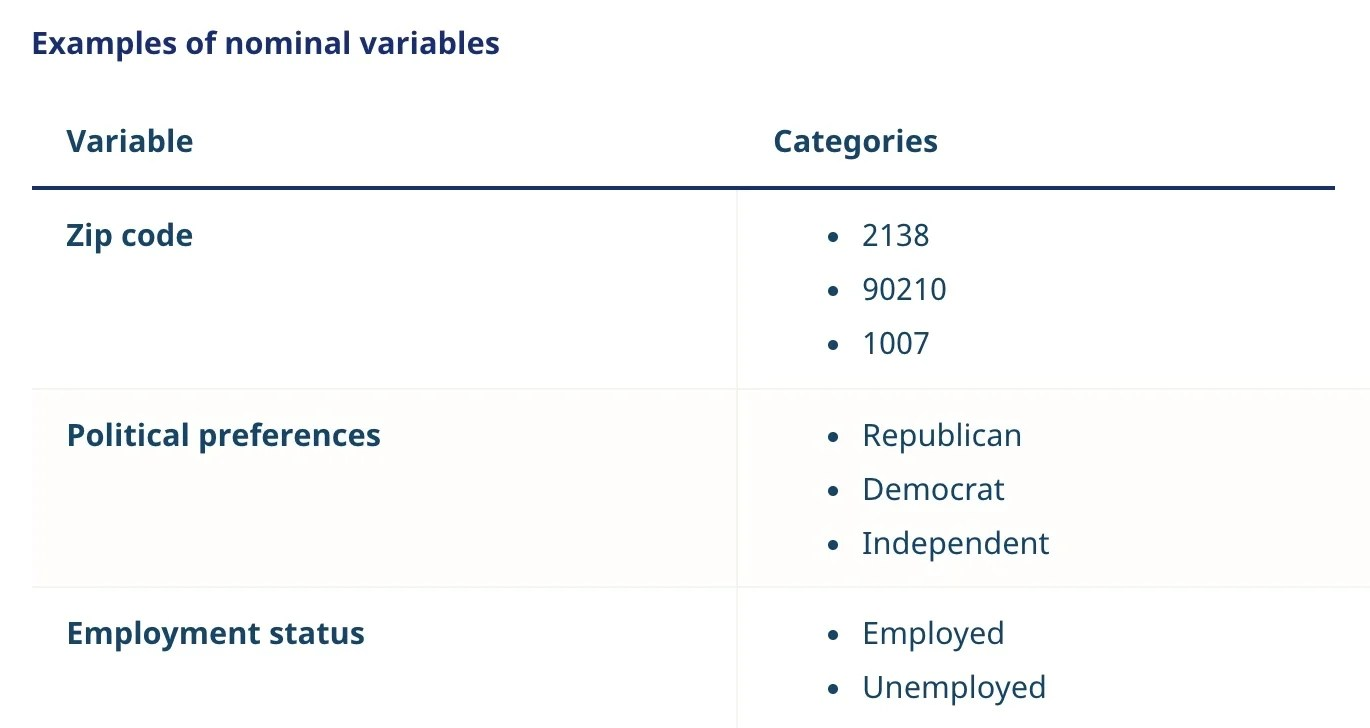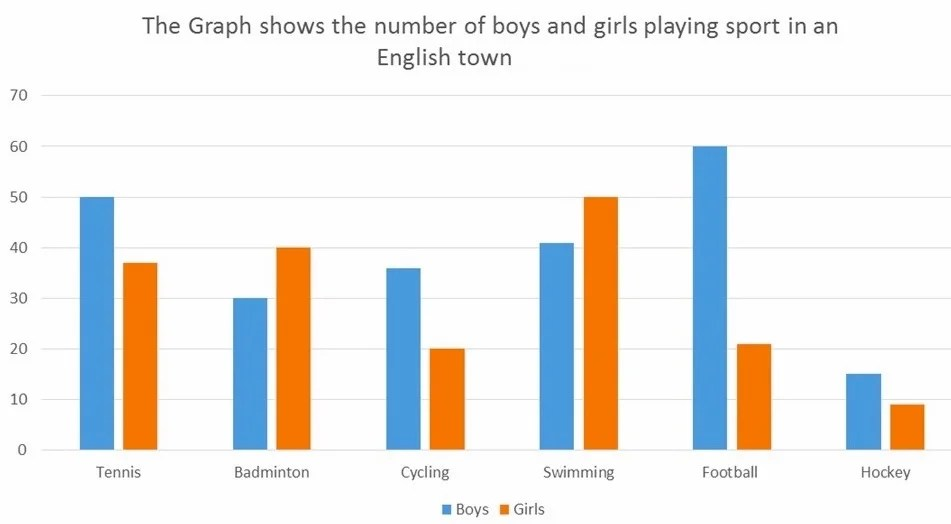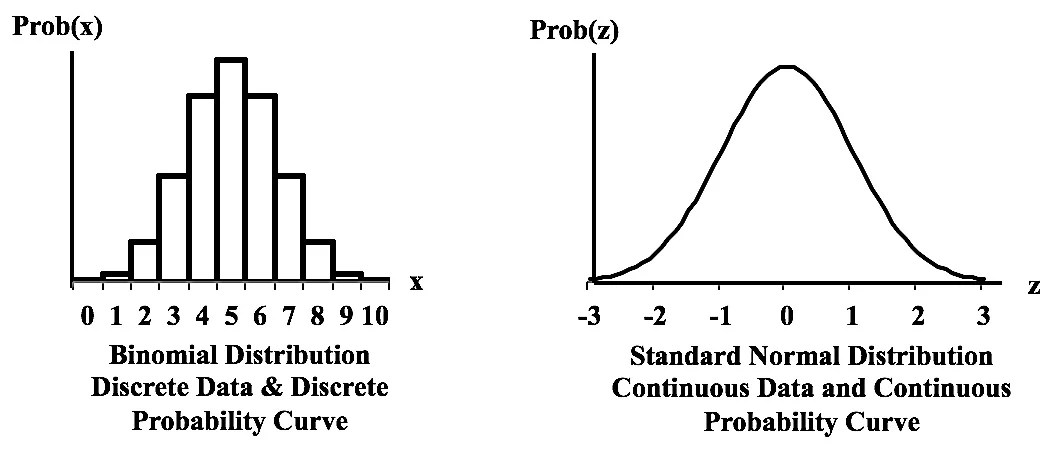# 4 Different Types Of Data [With Examples]

Data are a set of facts or values, such as words numbers, measurements, and descriptions of variables. The term “data” is generally considered plural. Its singular form is “datum,” which represents a single value of a single variable.

Data are used in almost all fields, including

• Finance (debt, interest rates),
• Business management (revenue, profit margins),
• Governance (literacy rates, unemployment rates),
• Non-profit organizations (number of homeless people), and
• Scientific research (observational, experimental, derived data).

There are literally countless forms of data. What’s interesting is that all these data can be split into two categories: quantitative and qualitative.

Quantitative Data

Quantitative data are information that can be measured using numbers and values. This type of data is mostly used for mathematical calculations and statistical analysis. It can be easily evaluated and verified using various mathematical techniques.

Quantitative data are generally concise and close-ended. They are often used to ask questions such as “how many” or “how much,” followed by conclusive information.

Simple examples of quantitative data include the number of tigers in a zoo, the weight of a person, the price of a product, and indoor temperature.

Qualitative Data

Qualitative data are non-statistical, which means they cannot be expressed in numbers. They can be semi-structured or unstructured.

Unlike quantitative data, qualitative data are open for exploration. They can be used to ask questions such as “why” or “how.” Data obtained from qualitative research are used for interpretations, theorizations, and building hypotheses, and initial understandings.

Simple examples of qualitative data include the color of the sky, names, smell, and ethnicity, such as Asian, African American.Quantitative and qualitative data can be further subdivided into four groups. To better explain these units of information, we have listed all four major types of data in statistics.

### 4. Ordinal DataType: Qualitative data

Ordinal data are categorical data where the values follow a natural order. The difference between the data values is somewhat meaningless or can’t be determined.

In ordinal scales, the order matters but not the difference between values.

Let’s consider a survey in which 100 people earning between \$30,000 and \$150,000 per annum were asked to rate their level of financial happiness.

A delivery guy making \$60,000 per year may be on a 9/10 scale, while a senior theoretical physicist earning \$100,000 rates 5/10. This shows that the scale is influenced by personal choices and not due to any predefined standard.

Key characteristics of ordinal data

• Ordinal values only show sequence
• Numbers can be assigned to ordinal data
• Mathematical calculations cannot be done with ordinal values
• The differences between ordinal numbers may or may not be equal

Generally, ordinal values are assessed via closed-ended surveys questions that give participants multiple possible answers to choose from. In social sciences, this type of data is collected using the Likert scale, which is made up of 3 or more Likert-type questions.

Examples of ordinal values include

• Social Economic Status (low income, middle income, high income)
• Satisfaction Rating (extremely unhappy, unhappy, neutral, happy, extremely happy)

Most companies utilize visualization tools to analyze ordinal data collected from their customers and employees. These tools represent data in tables where each row indicates a distinct category. Some present data in easy-to-understand bar charts and graphs.

### 3. Nominal DataType: Qualitative data

Nominal data are a group of non-parametric variables that are used for naming or labeling things. It is often called “named” data.

A nominal scale consists of a variable with categories that don’t have any order or ranking.  Although you can assign numbers to nominal variables if you want, the order will remain arbitrary and mathematical calculations (like mean, median, and standard deviation) would be meaningless.

For instance, very high, high, very low, low, medium are all nominal data when considered individually. However, when you put them on a scale and arrange them in a specific order (very high, high, medium, low, very low), they become ordinal data.

Typically, nominal data are collected via multiple-choice questions, open- and close- ended questions. For example,

• Which city are you from? (followed by a drop-down list of cities in the country)
• What is your field of study? (followed by a blank text box)

Key characteristics of nominal data

• Nominal data cannot be quantified
• They cannot be assigned a definite order
• Nominal data collection doesn’t include rating scales
• The mean and standard deviation cannot be established even if the data are arranged in order

The most effective way to analyze nominal data is the grouping technique, in which variables are clustered into groups, and for each group, the percentage or frequency can be calculated. It can also be presented in different visual forms, such as by using a bar or bubble chart.

Nominal data cannot be processed through mathematical operations, but you can use advanced statistical methods to analyze them in detail. The chi-squared test, for instance, determines whether there is a substantial difference between the estimated frequency and the observed frequency of the given values.

### 2. Discrete DataType: Quantitative data

Discrete data can take only certain values. These values are mostly positive integers and cannot be divided into smaller parts. For example, the number of employees working in a company is discrete data. You can’t count 21.5 or -21 employees.

Discrete data may contain an infinite number of values, but each is distinct and there is no other value in between. The value can either be numeric (such as numbers of devices) or categorical (such as male or female, red or blue, or true or false).

Characteristics of discrete data

• Discrete data can take only specific values
• Values can be counted
• Values cannot be divided into smaller pieces
• Data can be easily visualized and demonstrated

Discrete values do not necessarily have to be whole numbers. For example, you may have a shoe size of 8.5, which is a fixed value. You cannot buy a shoe of size 8.23.

An industry example of discrete data

Data from a washing machine test (pass or fail) can be collected to see whether the machine is ready for shipping. Engineers can analyze a particular machine by

• checking the number of times the machine test passed or failed
• making 20 additional tests over the course of two days

Based on results, engineers can classify each unit as pass (operable within a specific volt range) or fail.

Discrete data can be presented in various graphs. The bar graph is the most effective way to show discrete data as finite values can be displayed clearly through horizontal or vertical bars. The frequency table can also clearly represent discrete values through tally marks and the frequency of each variable.

### 1. Continuous DataType: Quantitative data

Continuous data can be measured on an infinite scale. It can take any value between two variables, no matter how small. For example, a person’s weight could be any value (within the range of human weights), not just a fixed digit. It could be an integer (75) or a decimal number (74.92).

Key characteristics of continuous data

• Continuous data contain random variables, which may or may not be integers.
• It can change over time and have different values at different time intervals.
• It can be measured via data analysis techniques, such as skews and line graphs.

Continuous data is far more descriptive than discrete data — it tells you a lot about data.

You can calculate the standard deviation (spread), the average (center), and measure the sharpness of the peak of a frequency-distribution curve (called kurtosis). Overall, you can summarize the data with descriptive statistics.

This level of detail is paramount for engineers, researchers, manufacturers, to name a few. Various industries and commercial entities use this kind of data due to their vast applications.

Continuous data makes it easier for businesses to precisely analyze their numbers by using only small or restricted samples. This information not only provides detailed insights into the multiple sources of variation but also helps businesses understand why key figures and statistics are changing.

In order to efficiently analyze continuous data, most companies utilize a method called regression analysis. It’s a reliable way of identifying which variables have the most impact on a topic of interest, how these variables influence each other, and which variables can be ignored.

##### How many data types are there in computer science?

In computer science and computer programming, variables are used to store numbers, text, and various other complicated types of data. Almost all computer programs, from music players and galleries to video games and social media apps, use these basic data types to represent all possible information:

• Integer: used for whole numbers
• Float: used for fractions or decimal points
• Character: used for single letters
• String: used for combinations of any character (numbers, letters, symbols)
• Boolean: used for yes/no or true/false options.
##### What’s the difference between structured and unstructured data?
 Structured Data Unstructured Data Clearly defined and easy to search and analyze Requires more work to process and understand Often stored in data warehouses Stored in data lakes Exists in predefined formats Often exists in various random formats Usually has a row-column format and very explicit metadata elements Not governed by strict rules or shared formats

There is another category called semi-structured data. As the name suggests, it’s a hybrid of structured and unstructured data. It lacks a fixed schema and contains crucial insights that can be hard to extract and process without an intelligent data governance strategy.

##### What are interval data and ratio data?

Both interval data and ratio data are measured along a scale, in which every point is placed at an equal distance from one another. For example, the data collected on a thermometer is interval data (because markings are equidistant).

On an interval scale, a variable can be negative too. Arithmetic operations can be performed, but they are limited to addition and subtraction only.

Unlike interval variables, ratio variables can have zero characteristics. The zero point makes it possible to measure multiple values and perform mathematical operations like multiplication and division. Age, weight, height, temperature (measured in Kelvin), and sales figures, are examples of ratio data.

Ratio variables are used to perform some of the most complex analyses, including TURF, SWOT, Cross-tabulation, and Conjoint.

##### Why is it important to classify a variable?

Data variables are classified to conduct statistical computations and analyses. Statistical algorithms provide businesses with more freedom to forecast results, especially for data that is versatile and sensitive in nature.

In order to use these algorithms, one must know what kind of data they are dealing with. For example, it wouldn’t make sense to calculate the average of names of the employees working in a company. You have to analyze optimal data differently than continuous data. Otherwise, it would result in incorrect analysis.Written by
###### Varun Kumar

Varun Kumar is a professional science and technology journalist and a big fan of AI, machines, and space exploration. He received a Master's degree in computer science from GGSIPU University. To find out about his latest projects, feel free to directly email him at [email protected]